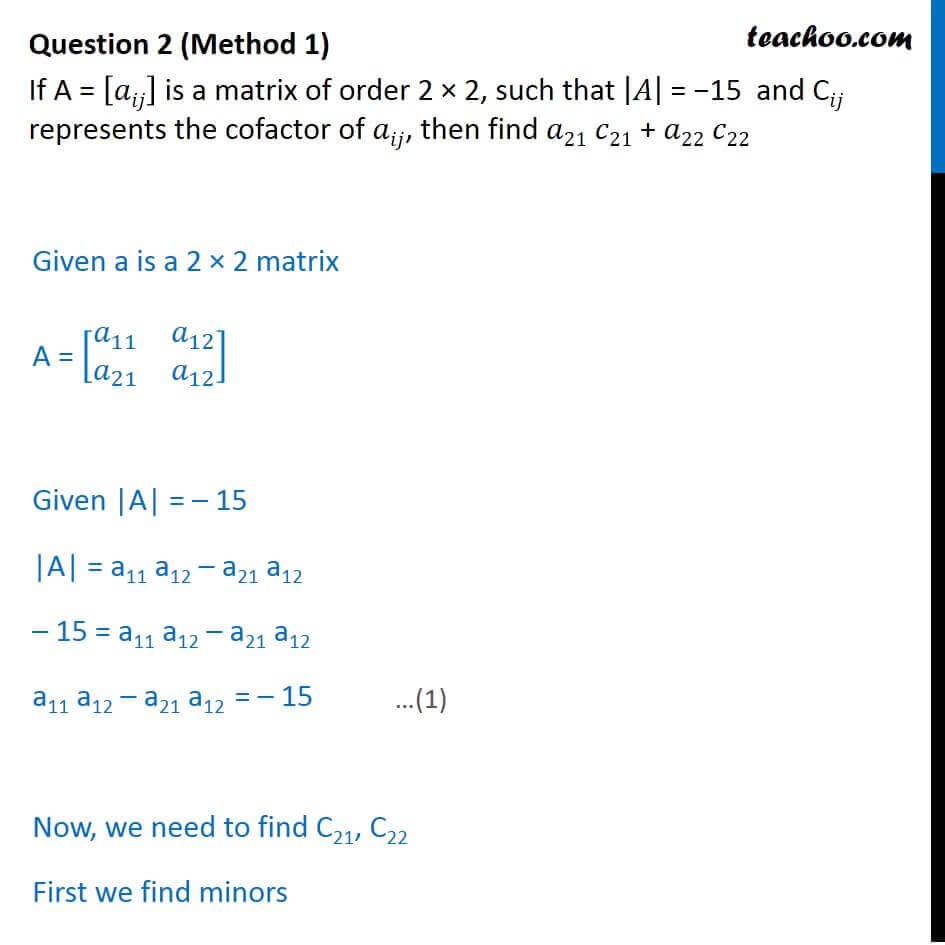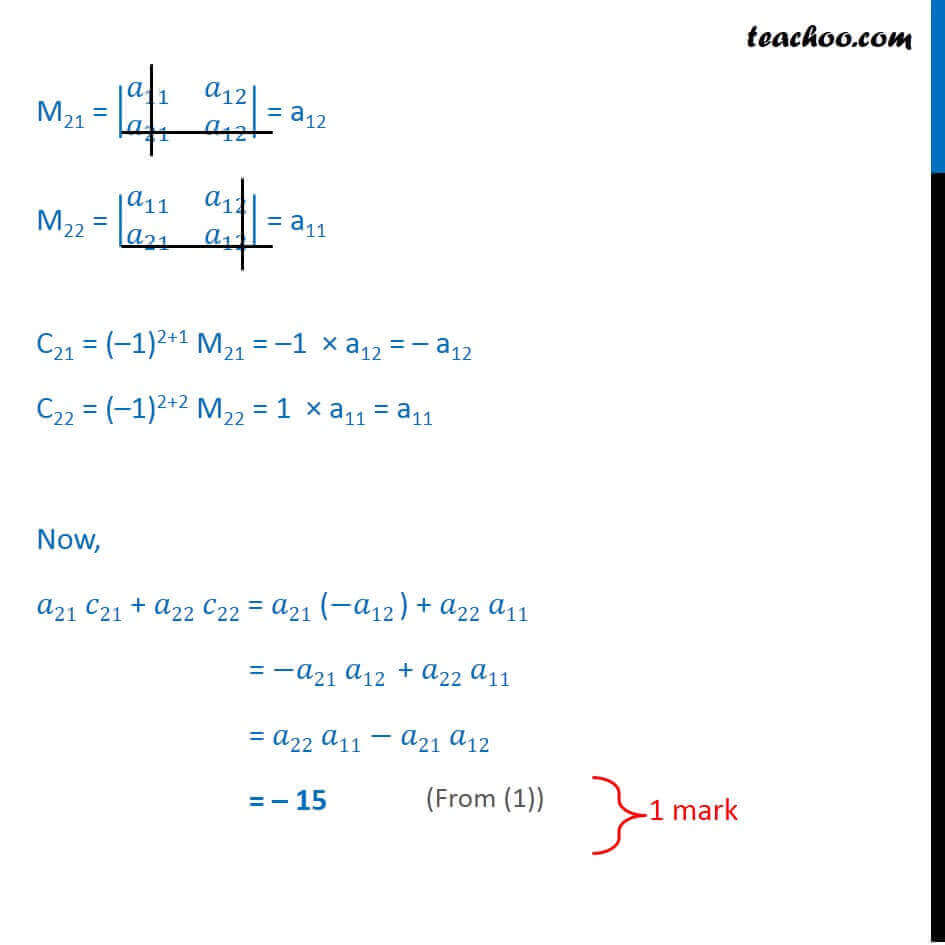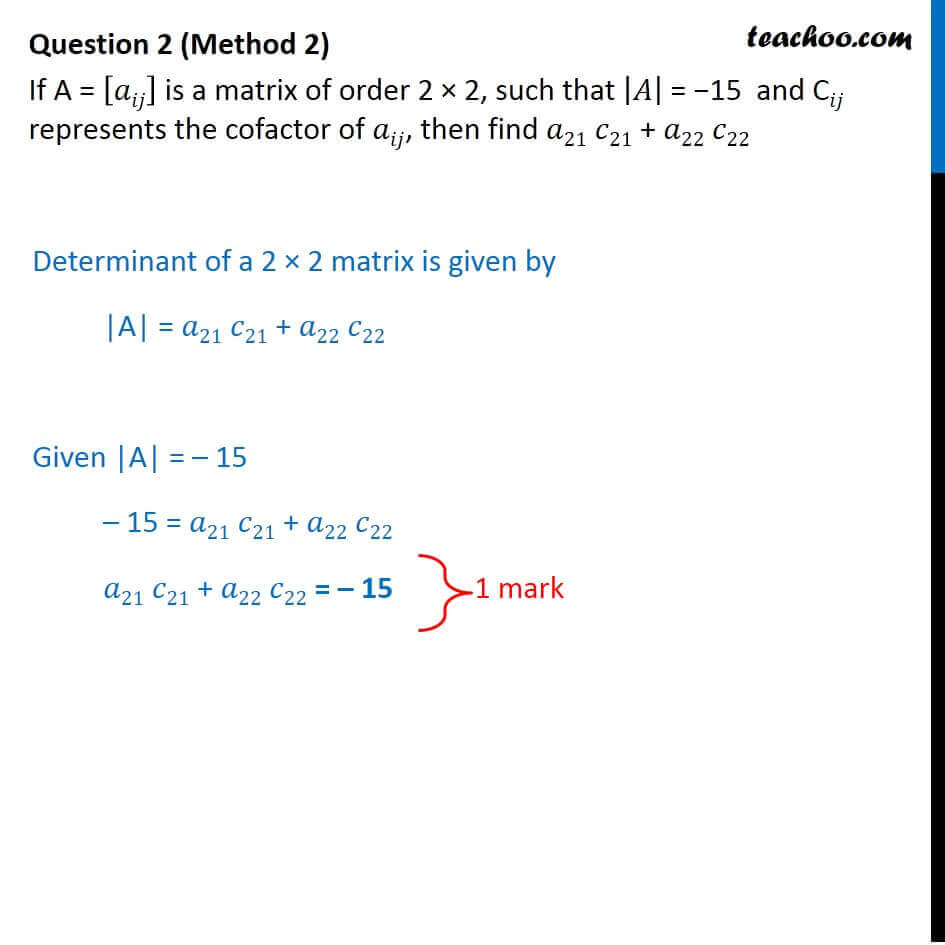CBSE Class 12 Sample Paper for 2018 Boards

Class 12
Solutions of Sample Papers and Past Year Papers - for Class 12 Boards

### If A = [a ij ] is a matrix of order 2 × 2, such that |A|  = −15  and C ij represents the cofactor of a ij , then find a 21 c 21 + a 22 c 22

This is a question of CBSE Sample Paper - Class 12 - 2017/18.Introducing your new favourite teacher - Teachoo Black, at only ₹83 per month

### Transcript

Question 2 (Method 1) If A = [𝑎𝑖𝑗] is a matrix of order 2 × 2, such that |𝐴| = −15 and C𝑖𝑗 represents the cofactor of 𝑎𝑖𝑗, then find 𝑎21 𝑐21 + 𝑎22 𝑐22 Given a is a 2 × 2 matrix A = [■8(𝑎_11&𝑎_12@𝑎_21&𝑎_12 )] Given |A| = – 15 |A| = a11 a12 – a21 a12 – 15 = a11 a12 – a21 a12 a11 a12 – a21 a12 = – 15 Now, we need to find C21, C22 First we find minors M21 = |■8(𝑎_11&𝑎_12@𝑎_21&𝑎_12 )| = a12 M22 = |■8(𝑎_11&𝑎_12@𝑎_21&𝑎_12 )| = a11 C21 = (–1)2+1 M21 = –1 × a12 = – a12 C22 = (–1)2+2 M22 = 1 × a11 = a11 Now, 𝑎21 𝑐21 + 𝑎22 𝑐22 = 𝑎21 (−𝑎12 ) + 𝑎22 𝑎11 = −𝑎21 𝑎12 + 𝑎22 𝑎11 = 𝑎22 𝑎11 − 𝑎21 𝑎12 = – 15 Question 2 (Method 2) If A = [𝑎𝑖𝑗] is a matrix of order 2 × 2, such that |𝐴| = −15 and C𝑖𝑗 represents the cofactor of 𝑎𝑖𝑗, then find 𝑎21 𝑐21 + 𝑎22 𝑐22 Determinant of a 2 × 2 matrix is given by |A| = 𝑎21 𝑐21 + 𝑎22 𝑐22 Given |A| = – 15 – 15 = 𝑎21 𝑐21 + 𝑎22 𝑐22 𝑎21 𝑐21 + 𝑎22 𝑐22 = – 15